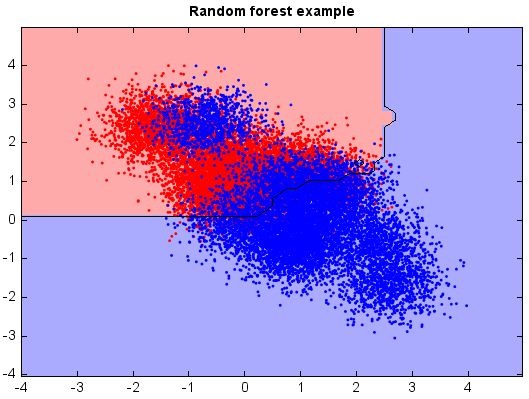# Random forest¶

Random forest is an ensemble classifier that consists of many decision trees and outputs the majority vote of individual trees. The method combines bagging idea and the random selection of features.

Each tree is constructed using the following algorithm:

• If the number of cases in the training set is N, randomly sample N cases with replacement from the original data. This sample will be the training set for growing the tree.

• If there are M input variables, a number m << M is specified such that at each node, m variables are selected at random out of the M and the best split on these m is used to split the node. The value of m is held constant during the forest growing.

• Each tree is grown to the largest extent possible. There is no pruning.

```from miml import datasets
from miml.classification import RandomForest

fn = os.path.join(datasets.get_data_home(), 'classification', 'toy',
'toy-test.txt')
format='%2f', index_col=0)

X = df.values
y = array(df.index.data)

model = RandomForest(ntrees=100, max_nodes=200)
model.fit(X, y)

# Plot the decision boundary. For that, we will assign a color to each
# point in the mesh [x_min, x_max]x[y_min, y_max].
x_min, x_max = X[:, 0].min() - 1, X[:, 0].max() + 1
y_min, y_max = X[:, 1].min() - 1, X[:, 1].max() + 1
n = 50  # size in the mesh
xx, yy = np.meshgrid(np.linspace(x_min, x_max, n),
np.linspace(y_min, y_max, n))
data = np.vstack((xx.flatten(), yy.flatten())).T
Z = model.predict(data)

# Put the result into a color plot
Z = Z.reshape(xx.shape)

#Plot
# Create color maps
cmap_light = ['#FFAAAA', '#AAAAFF']
cmap_bold = ['#FF0000', '#0000FF']
imshow(xx[0,:], yy[:,0], Z, colors=cmap_light)
# Plot also the training points
ls = plt.scatter(X[:, 0], X[:, 1], c=y,
edgecolor=None, s=3, levels=[0,1], colors=cmap_bold)
plt.contour(xx[0,:], yy[:,0], Z, [0.5], color='k', smooth=False)
plt.xlim(xx.min(), xx.max())
plt.ylim(yy.min(), yy.max())
plt.title("Random forest example")
```